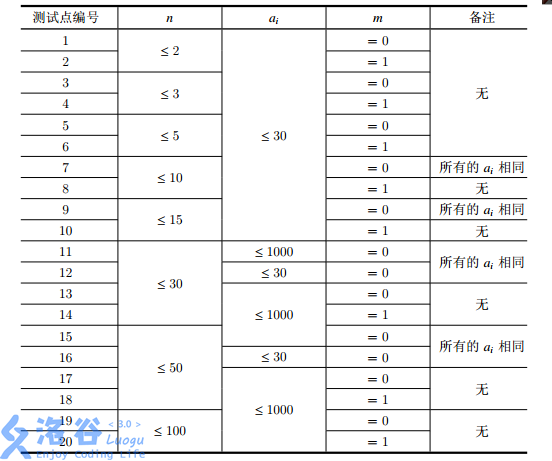# P3749 [六省联考2017]寿司餐厅

• 455通过
• 923提交
• 评测方式 云端评测
• 标签 广度优先搜索,BFS 最大流 最小割 深度优先搜索,DFS 各省省选 2017 高性能
• 难度 省选/NOI-
• 时空限制 1000ms / 128MB
• 提示：收藏到任务计划后，可在首页查看。

## 题目描述

Kiana 最近喜欢到一家非常美味的寿司餐厅用餐。

每天晚上，这家餐厅都会按顺序提供 $n$ 种寿司，第 $i$ 种寿司有一个代号 $a_i$ 和美味度 $d_{i, i}$，不同种类的寿司有可能使用相同的代号。每种寿司的份数都是无限的，Kiana 也可以无限次取寿司来吃，但每种寿司每次只能取一份，且每次取走的寿司必须是按餐厅提供寿司的顺序连续的一段，即 Kiana 可以一次取走第 $1, 2$ 种寿司各一份，也可以一次取走第 $2, 3$ 种寿司各一份，但不可以一次取走第 $1, 3$ 种寿司。

由于餐厅提供的寿司种类繁多，而不同种类的寿司之间相互会有影响：三文鱼寿司和鱿鱼寿司一起吃或许会很棒，但和水果寿司一起吃就可能会肚子痛。因此，Kiana 定义了一个综合美味度 $d_{i, j} \ (i < j)$，表示在一次取的寿司中，如果包含了餐厅提供的从第 $i$ 份到第 $j$ 份的所有寿司，吃掉这次取的所有寿司后将获得的额外美味度。由于取寿司需要花费一些时间，所以我们认为分两次取来的寿司之间相互不会影响。注意在吃一次取的寿司时，不止一个综合美味度会被累加，比如若 Kiana 一次取走了第 $1, 2, 3$ 种寿司各一份，除了 $d_{1, 3}$ 以外，$d_{1, 2}, d_{2, 3}$ 也会被累加进总美味度中。

神奇的是，Kiana 的美食评判标准是有记忆性的，无论是单种寿司的美味度，还是多种寿司组合起来的综合美味度，在计入 Kiana 的总美味度时都只会被累加一次。比如，若 Kiana 某一次取走了第 $1, 2$ 种寿司各一份，另一次取走了第 $2, 3$ 种寿司各一份，那么这两次取寿司的总美味度为 $d_{1, 1} + d_{2, 2} + d_{3, 3} + d_{1, 2} + d_{2, 3}$，其中 $d_{2, 2}$ 只会计算一次。

奇怪的是，这家寿司餐厅的收费标准很不同寻常。具体来说，如果 Kiana 一共吃过了 $c \ (c > 0)$ 代号为 $x$ 的寿司，则她需要为这些寿司付出 $mx^2 + cx$ 元钱，其中 $m$ 是餐厅给出的一个常数。

现在 Kiana 想知道，在这家餐厅吃寿司，自己能获得的总美味度（包括所有吃掉的单种寿司的美味度和所有被累加的综合美味度）减去花费的总钱数的最大值是多少。由于她不会算，所以希望由你告诉她。

## 输入输出格式

输入格式：

第一行包含两个正整数 $n, m$，分别表示这家餐厅提供的寿司总数和计算寿司价格中使用的常数。
第二行包含 $n$ 个正整数，其中第 $k$ 个数 $a_k$ 表示第 $k$ 份寿司的代号。
接下来 $n$ 行，第 $i$ 行包含 $n - i + 1$ 个整数，其中第 $j$ 个数 $d_{i, i+j-1}$ 表示吃掉寿司能获得的相应的美味度，具体含义见问题描述。

输出格式：

输出共一行包含一个正整数，表示 Kiana 能获得的总美味度减去花费的总钱数的最大值。

## 输入输出样例

输入样例#1： 复制
3 1
2 3 2
5 -10 15
-10 15
15
输出样例#1： 复制
12
输入样例#2： 复制
5 0
1 4 1 3 4
50 99 8 -39 30
68 27 -75 -32
70 24 72
-10 81
-95
输出样例#2： 复制
381
输入样例#3： 复制
10 1
5 5 4 4 1 2 5 1 5 3
83 91 72 29 22 -5 57 -14 -36 -3
-11 34 45 96 32 73 -1 0 29
-48 68 44 -5 96 66 17 74
88 47 69 -9 2 25 -49
86 -9 -77 62 -10 -30
2 40 95 -74 46
49 -52 2 -51
-55 50 -44
72 22
-68
输出样例#3： 复制
1223

## 说明

【样例1说明】

在这组样例中，餐厅一共提供了3份寿司，它们的代号依次为a1=2，a2=3，a3=2，计算价格时的常数m=1。在保证每次取寿司都能获得新的美味度的前提下，Kiana一共有14种不同的吃寿司方案：

1.Kiana一个寿司也不吃，这样她获得的总美味度和花费的总钱数都是0，两者相减也是0；

2.Kiana只取1次寿司，且只取第1个寿司，即她取寿司的情况为{[1,1]}，这样获得的总美味度为5，花费的总钱数为1-2^2+1*2=6，两者相减为-1；

3.Kiana只取1次寿司，且只取第2个寿司，即她取寿司的情况为{[2,2]}，这样获得的总美味度为-10，花费的总钱数为1-3^2+1*3=12，两者相减为-22；

4.Kiana只取1次寿司，且只取第3个寿司，即她取寿司的情况为{[3,3]}，这样获得的总美味度为15，花费的总钱数为1*2^2+1*2=6，两者相减为9；

5.Kiana只取1次寿司，且取第1,2个寿司，即她取寿司的情况为{[1,2]}，这样获得的总美味度为5+(-10)+(-10)=-1

5，花费的总钱数为(1-2^2+1*2)+(1-3^2+1*3)=18，两者相减为-33；

6.Kiana只取1次寿司，且取第2,3个寿司，即她取寿司的情况为{[2,3]}，这样获得的总美味度为(-10)+15+15=20，花费的总钱数为(1-2^2+1*2)+(1*3^2+1*3)=18，两者相减为2；

7.Kiana只取1次寿司，且取第1,2,3个寿司，即她取寿司的情况为{[1,3]}，这样获得的总美味度为5+(-10)+15+(-10)+15+15=30，花费的总钱数为(1*2^2+2*2)+(1*3^2+1*3)=20，两者相减为10。

8.Kiana取2次寿司，第一次取第1个寿司，第二次取第2个寿司，即她取寿司的情况为{[1,1],[2,2]}，这样获得的总美味度为5+(-10)=-5，花费的总钱数为(1*2^2+1*2)+(1*3^2+1*3)=18，两者相减为-23；

9.Kiana取2次寿司，第一次取第1个寿司，第二次取第3个寿司，即她取寿司的情况为{[1,1],[3,3]}，这样获得的总美味度为5+15=20，花费的总钱数为1*2^2+2*2=8，两者相减为12；

10.Kiana取2次寿司，第一次取第2个寿司，第二次取第3个寿司，即她取寿司的情况为{[2,2],[3,3]}，这样获得的总美味度为(-10)+15=5，花费的总钱数为(1*2^2+1*2)+(1*3^2+1*3)=18，两者相减为-13；

11.Kiana取2次寿司，第一次取第1,2个寿司，第二次取第3个寿司，即她取寿司的情况为{[1,2],[3,3]}，这样获得的总美味度为5+(-10)+(-10)+15=0，花费的总钱数为(1*2^2+2*2)+(1*3^2+1*3)=20，两者相减为-20；

[下转输出格式]提示
标程仅供做题后或实在无思路时参考。
请自觉、自律地使用该功能并请对自己的学习负责。
如果发现恶意抄袭标程，将按照I类违反进行处理。